# Energy Recycling in DC Motor Drives

### Introduction

When decelerating a moving mass in a permanent magnet motor drive, energy stored in the mechanical system may be returned through the motor driver to the power source. If this energy is not properly accounted for, it can cause damage to the motor driver or the rest of the system by causing an increase in the power supply voltage.

In this article, we will look at ways to dissipate this energy safely. To simplify the examples, a DC brush motor is shown. This applies to brushless motor systems as well.

### Conservation of Energy

The conservation of energy is a basic principle of physics—energy cannot be created nor destroyed.

When something (such as a mass) is moving or rotating, it accumulates kinetic energy. In a motor system, that kinetic energy comes from a power source that provides electrical energy to a motor, which creates torque to accelerate the mass.

There is energy stored both in the inertia of the motor’s rotor and also in the mechanical system attached to the motor. For simplicity, envision the mechanical system as a flywheel coupled to the motor shaft (see Figure 1).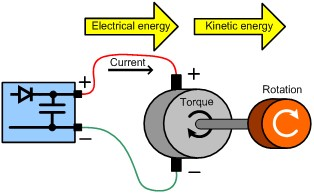Figure 1: Flywheel Example of a Mechanical System

The kinetic energy can be calculated with ½ Iω2, where I is the moment of inertia and ω is the angular velocity. The higher the speed or the more inertia there is, the more energy is stored.

This is a very obvious concept—it takes energy to get something moving. What is less obvious is what happens when you want to stop the motion. To stop or slow a moving mass, the stored kinetic energy has to go somewhere. But where?

When you disconnect the power from a spinning motor, the energy stored in the moving mass is dissipated into the mechanical losses in the system. Most of this energy is turned into heat due to friction (see Figure 2). Unless there is a great deal of friction, the motor coasts to a stop very slowly. The motor turns into a generator, but since there is no path for current to flow, there is no electromagnetic torque to help stop the motor.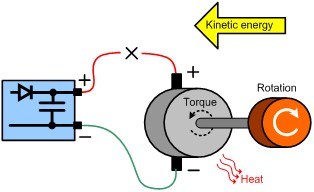Figure 2: Friction in a Stopping Motor

If a path is provided for current to flow from this generator by shorting the output of the motor, the current generates a torque in opposition to the direction of rotation (see Figure 3). This causes the motor to come to a stop quickly. In this case, the energy is mostly dissipated as heat in the winding resistance of the motor and also in any resistance in the current path, shorting the motor.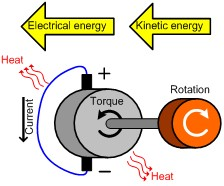Figure 3: Torque in Opposition to Rotation

This is sometimes called a “short brake.” In reality, a short circuit is usually applied by turning on the low-side MOSFETs of an H-bridge to provide a current path.

When a control system wants to decrease the speed of the motor quickly, the polarity of the current applied to the motor is reversed to provide a torque in opposition to the motion. When this is done, the stored kinetic energy can be driven back through the motor driver circuit into the power supply.

If the power source were a perfect battery, then energy would flow back into the battery and be recycled. However, in the real world, the power source is usually a DC power supply, and unless this supply is specially designed, a DC power supply can only source current. Because it cannot sink current, the only place the energy has to go is into the capacitance that is part of the power supply.

The amount of energy stored in a capacitor can be calculated with ½ CV2, where C is the capacitance and V is the voltage. The voltage across the capacitor must increase as energy flows into it (see Figure 4).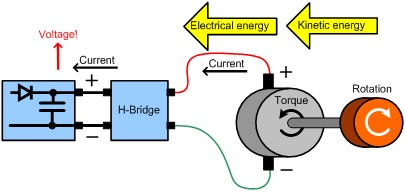Figure 4: Increasing Capacitor Voltage with Increasing Energy

If the amount of energy is small (either the speed is low or the inertia is small), then the voltage increase may be small enough that it will not cause any problems. However, in some cases, if there is too much energy or not enough capacitance, the voltage may rise to destructive levels. This can damage the motor driver circuit or other circuitry connected to the same power supply.

### Dissipating the Energy

There are a few ways we can deal with the energy that is being recycled back to the power source. One is to place a large amount of capacitance on the power supply. In some cases, this may be all that is needed, but in most cases, large capacitors are not practical due to physical or cost constraints.

Another way to deal with the energy is to use a semiconductor clamp device across the power supply, such as a TVS or Zener diode (see Figure 5). The clamp is designed to break down at just above the normal operating voltage of the power supply. When recycled energy causes the voltage to rise, the clamp breaks down and protects the system. The energy returned to the power supply is dissipated as heat in the clamp device.

If the amount of energy is modest, this solution is simple and easy.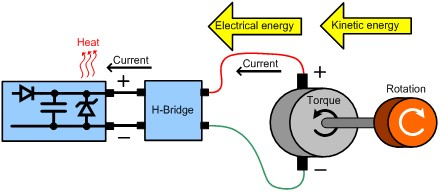Figure 5: Semiconductor Clamp Used to Dissipate Energy

In larger systems, use a simple clamp often is not practical, since the amount of energy that needs to be dissipated is too great. In these cases, an active clamp circuit can be used to dissipate the energy into a resistive load.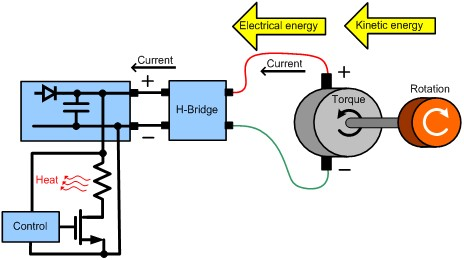Figure 6: Active Circuit Clamp Used to Dissipate Energy

The clamp circuit works by monitoring the supply voltage using a comparator or similar circuitry (see Figure 6). If the voltage exceeds a preset threshold (just above the normal operating voltage), a load resistor is switched across the supply to dissipate the energy.

### Conclusion

This article provided a high-level overview of how energy can be fed back from a motor to a power source from a mechanical system, and how it can be dealt with in motor drive electronics. The math required to determine the component values for a given system properly is beyond the scope of this article, but more details, including calculations for capacitance and clamp components, are provided in the application note AN132 “Input Capacitor and Over-Voltage Protection Circuit Design” available at www.monolithicpower.com.

__________________________________________